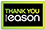# Bayesian methods in statistics

PAPERBACK
This book walks you through learning probability and statistics from a Bayesian point of view.  From an introduction to probability theory through to frameworks for doing rigorous calculations of probability, it discusses Bayes' Theorem before illustrating how to use it in a variety of different situations with data addressing social and psychological issues. The book also: Equips you with coding skills in the statistical modelling language Stan and programming language R. Discusses how Bayesian approaches to statistics compare to classical approaches. Introduces Markov Chain Monte Carlo methods for doing Bayesian statistics through computer simulations, so you understand how Bayesian solutions are implemented. Features include an introduction to each chapter and a chapter summary to help you check your learning. All the examples and data used in the book are also available in the online resources so you can practice at your own pace. For readers with some understanding of basic mathematical functions and notation, this book will get you up and running so you can do Bayesian statistics with confidence.
€51.79155 Reward Points
In stock online
Delivery in 2-3 working days

Any purchases for more than €10 are eligible for free delivery anywhere in the UK or Ireland!

This book walks you through learning probability and statistics from a Bayesian point of view.  From an introduction to probability theory through to frameworks for doing rigorous calculations of probability, it discusses Bayes' Theorem before illustrating how to use it in a variety of different situations with data addressing social and psychological issues. The book also: Equips you with coding skills in the statistical modelling language Stan and programming language R. Discusses how Bayesian approaches to statistics compare to classical approaches. Introduces Markov Chain Monte Carlo methods for doing Bayesian statistics through computer simulations, so you understand how Bayesian solutions are implemented. Features include an introduction to each chapter and a chapter summary to help you check your learning. All the examples and data used in the book are also available in the online resources so you can practice at your own pace. For readers with some understanding of basic mathematical functions and notation, this book will get you up and running so you can do Bayesian statistics with confidence.
Quantity:
In stock online
Delivery in 2-3 working days155 Reward Points

Any purchases for more than €10 are eligible for free delivery anywhere in the UK or Ireland!

€51.79
In stock online
Delivery in 2-3 working days